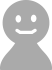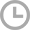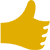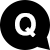# 小数点以下はどこまで計算すればよいでしょうかって英語でなんて言うの？( NO NAME )
2018/02/14 10:54417540

2018/02/20 21:33Decimal places is the key term here. Decimal places are "rounded off" when the amount of numerals after the decimal point are limited.
"Decimal places"（少数位）がここでは重要な語となります。 "Decimal places"とは小数点以下の数字が 決まっていたら"rounded off"（切り捨てる） と言う意味になります。2018/03/03 00:22Example "should I calculate 2 places after the decimal point?". "How many places after the decimal point should I calculate?".2018/03/03 02:18A 'decimal place' is the position of a number digit to the right of a decimal point. For example: "Calculate to three decimal places".
decimal place(少数位)は、decimal point(小数点)の右の数字の数です。例えば 例文 "Calculate to three decimal places". 小数点第3位まで計算しなさい。
2018/03/21 23:56You can talk in terms of 'decimal places' or in terms of 'decimal points.' They are the same thing. You may be given an instruction: "Please work to two decimal places and round up or down as necessary." To round up or down = This means the third decimal point would be ignored if 0.4 or below, or if 0.5 or above, the second decimal figure would go up by one in this case.

2018/03/06 21:45"How many places after the decimal points must I work out to?" This asks the teacher how many numbers after the decimal point you need. "How many places after the decimal points do I need to calculate?" This is another way of asking how many numbers you need to work out after the point.

2018/03/08 21:32I should calculate to how many decimal places?/I'm rounding off to how many decimal places?- A decimal numer is a fraction and not a whole number like 34.45 ml, 1.58cm A:I'm rounding off to how many decimal places. B: two decimal places for all questions.

2018/03/27 21:03"Within how many decimal points"? will determine just how accurate the maths question will be answered... in percentages of "a whole number" ;-D Its a complex calculation...within how many decimal points should I answer ?
"Within how many decimal points?" （小数点以下どこまでですか？） これは、算数の問題でどこまでこたえなくてはいけないか、 と言う意味になります。 "a whole number"（整数）の何パーセントかと言う事ですよね ;-D 【例】 Its a complex calculation...within how many decimal points should I answer ? （これは複雑な計算です、小数点以下どこまでこたえなくてはいけませんか？）
2022/05/25 19:22When talking about, "to round up," to a certain decimal, we are referring at what point can we cut off the number and start doing our equation or calculation. We can also use the verb phrases, "to need," and, "to have to," to talk about what is obligatory or not.417540•役に立った：4

•PV：17540

アンカーランキング
• 週間
• 月間
• 総合
メニュー